Basics of Geometry
edHelper subscribers - Create a new printable

Number of Keys
 Select the number of different printables: 1 key 2 keys 3 keys 4 keys 5 keys

Number of Pages (for each key)
 Select the number of pages: 1 page 2 pages 3 pages 4 pages

Include an answer key (answer keys will be at the end of the printable)
High School Geometry
High School Geometry
Math

 Name _____________________________Date ___________________ (Key 1 - Answer ID # 0465111)
1.

Victoria slices a pumpkin pie into equal sectors through the center of the pie. There are 10 slices. If 6 of the slices are removed, what angle do the remaining slices make? If necessary, round your answer to the nearest hundredth of a degree.

2.

Use each of these terms in a sentence, but your sentence cannot be the definition of the term. Make a little sketch that illustrates the use of the term for each sentence: acute angle, obtuse angle, right angle, straight angle, reflex angle.

3.

Two angles are supplements. The larger angle is 10more than three times the smaller. Find the measures of these two angles.

4.

Is it possible for two angles located on different parts of the surface of the earth to be complementary? Explain.

5.

Savannah said, "If you know one vertical angle, you know three other angles." What did she mean by this?

6.

How can you remember the difference between complements and supplements?

7.

Is it possible for two identical angles located at different places on the earth to be vertical angles? Explain.

8.

B is in the interior of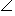ADC. If mADC = (7x - 73), mADB = (3x - 13), and mBDC = (2x - 18), find the value of x.

9.

You are presented with a coordinate axis system (x- and y-axes with center at the origin). There are two points, one at (6, 1) and another at (-1, 8). There is a line passing through each point, and each line is perpendicular to the other. One of these lines is horizontal, and the other is vertical. What two possible points identify the vertex of a right angle made by the two lines?

10.

Given:
D is in the interior ofABE.
E is in the interior ofDBF.
F is in the interior ofEBC.
mABC = 150, mEBC = 94,
mABD = mEBF, mFBC = 2mDBE
Which of the following is incorrect?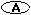mABD = 18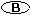mFBC = 76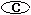mDBF = 56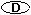mABF = 94Basics of Geometry
edHelper subscribers - Create a new printable

Number of Keys
 Select the number of different printables: 1 key 2 keys 3 keys 4 keys 5 keys

Number of Pages (for each key)
 Select the number of pages: 1 page 2 pages 3 pages 4 pages